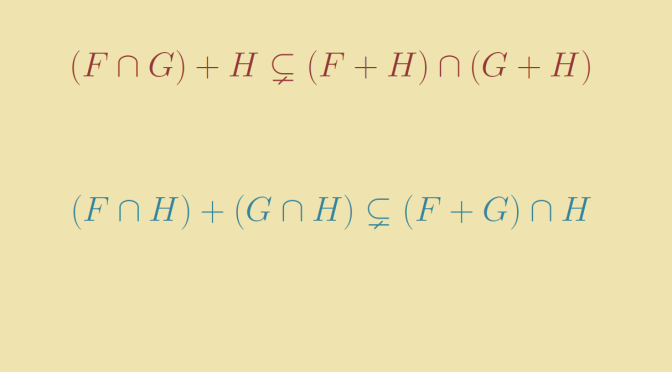Intersection and sum of vector subspaces

Let’s consider a vector space $$E$$ over a field $$K$$. We’ll look at relations involving basic set operations and sum of subspaces. We denote by $$F, G$$ and $$H$$ subspaces of $$E$$.

The relation $$(F \cap G) + H \subset (F+H) \cap (G + H)$$

This relation holds. The proof is quite simple. For any $$x \in (F \cap G) + H$$ there exists $$y \in F \cap G$$ and $$h \in H$$ such that $$x=y+h$$. As $$y \in F$$, $$x \in F+H$$ and by a similar argument $$x \in F+H$$. Therefore $$x \in (F+H) \cap (G + H)$$.

Is the inclusion $$(F \cap G) + H \subset (F+H) \cap (G + H)$$ always an equality? The answer is negative. Take for the space $$E$$ the real 3 dimensional space $$\mathbb R^3$$. And for the subspaces:

• $$H$$ the plane of equation $$z=0$$,
• $$F$$ the line of equations $$y = 0, \, x=z$$,
• $$G$$ the line of equations $$y = 0, \, x=-z$$,

We have $$F+H=G+H=E$$, $$(F \cap G)=\{0\}$$ which leads to $H = (F \cap G) + H \subsetneq (F+H) \cap (G + H) = E.$

The relation $$(F \cap H) + (G \cap H) \subset (F+G) \cap H$$

If $$f+g \in (F \cap H) + (G \cap H)$$ with $$f,g \in H$$ then $$f+g \in F+G$$ and $$f+g \in H$$ (as $$H$$ is a subspace). Hence the inclusion.

Again the inclusion can be strict. In particular this is the case if $$F, G, H$$ are 3 distinct lines of the plane $$\mathbb R^2$$. In that case $$F \cap H = G \cap H= \{0\}$$ while $$(F+G) \cap H = H$$.